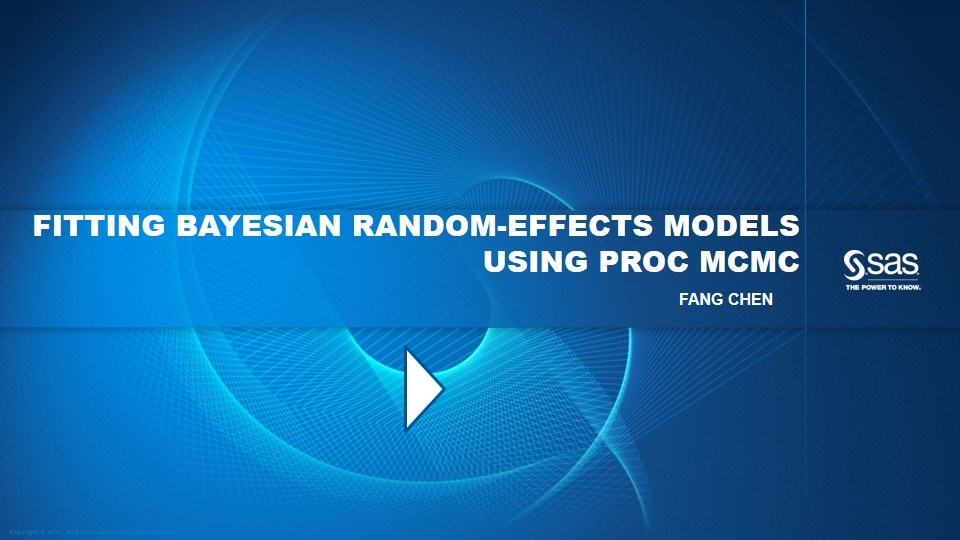# The MCMC Procedure

The MCMC procedure is a flexible, general-purpose Markov chain Monte Carlo simulation procedure that is suitable for fitting a wide range of Bayesian models. To use the procedure, you specify a likelihood function for the data and a prior distribution for the parameters. If you are fitting hierarchical models, you can also specify hyperprior distributions or you can specify random effects and their prior distributions. PROC MCMC obtains samples from the corresponding posterior distributions, produces summary and diagnostic statistics, and saves the posterior samples in an output data set that can be used for further analysis. You can analyze data that have any likelihood, prior, or hyperprior with PROC MCMC, as long as these functions can be programmed by using the SAS DATA step functions. The parameters can enter the model in any linear or nonlinear functional form.

### Sampling Algorithms

See the section "Sampling Methods" in the chapter "The MCMC Procedure" of the SAS/STAT® User's Guide.

### Priors

See the section "Standard Distributions" in the chapter "The MCMC Procedure" of the SAS/STAT® User's Guide for the distributions that PROC MCMC recognizes. PROC MCMC also enables you to specify other distributions that are not included in the list of standard distributions, as explained in the section "Specifying a New Distribution".

### VideosGetting Started with the MCMC Procedure By Fang Chen Air date: November 26, 2013Air date: December 12, 2011

### Examples

• Bayesian Zero-Inflated Poisson Regression
PDF   |   HTML

• Bayesian Exponential Mixture Model
PDF   |   HTML

• Bayesian Linear Regression with Standardized Covariates
PDF   |   HTML

• Bayesian Hierarchical Poisson Regression Model for Overdispersed Count Data Using SAS/STAT 9.2
PDF   |   HTML

• Bayesian Hierarchical Poisson Regression Model for Overdispersed Count Data Using SAS/STAT 9.3
PDF   |   HTML

• Bayesian Binomial Model with Power Prior Using the MCMC Procedure
PDF   |   HTML

• Bayesian Multivariate Prior for Multiple Linear Regression Using SAS/STAT 9.2
PDF   |   HTML

• Bayesian Multivariate Prior for Multiple Linear Regression Using SAS/STAT 9.3
PDF   |   HTML

• Bayesian Multinomial Model for Ordinal Data Using SAS/STAT 9.2
PDF   |   HTML

• Bayesian Multinomial Model for Ordinal Data Using SAS/STAT 9.3
PDF   |   HTML

• Bayesian Quantile Regression
PDF   |   HTML

• Bayesian LASSO
PDF   |   HTML

• Stochastic Search Variable Selection with PROC MCMC
PDF   |   HTML